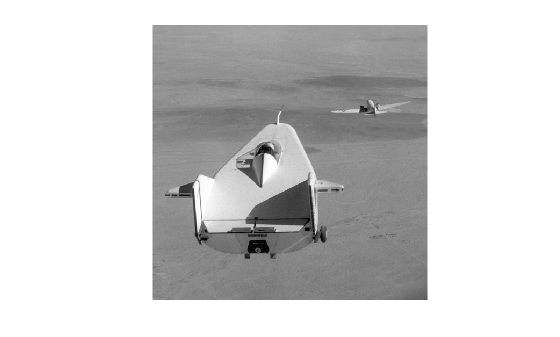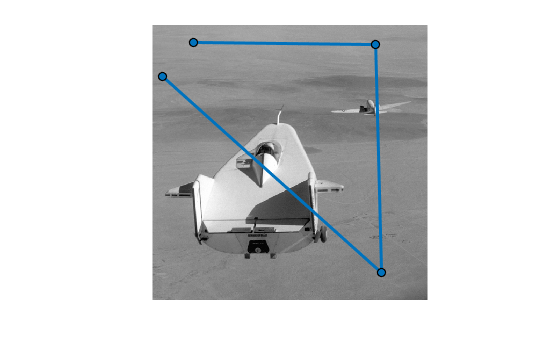# improfile

Pixel-value cross-sections along line segments

## Syntax

``improfile``
``improfile(n)``
``improfile(I,xi,yi)``
``improfile(I,xi,yi,n)``
``c = improfile(___)``
``[cx,cy,c] = improfile(I,xi,yi,n)``
``[cx,cy,c,xi,yi] = improfile(I,xi,yi,n)``
``[___] = improfile(x,y,I,xi,yi)``
``[___] = improfile(x,y,I,xi,yi,n)``
``[___] = improfile(___,method)``

## Description

````improfile` retrieves the intensity values of pixels along a line or a multiline path in the grayscale, binary, or RGB image in the current axes and displays a plot of the intensity values. If the specified path consists of a single line segment, `improfile` creates a two-dimensional plot of intensity values versus the distance along the line segment. If the path consists of two or more line segments, `improfile` creates a three-dimensional plot of the intensity values versus their x- and y-coordinates.With this syntax, you specify the line or path using the mouse, by clicking points in the image. Press Backspace or Delete to remove the previously selected point. To finish selecting points, adding a final point, press shift-click, right-click, or double-click. To finish selecting points without adding a final point, press Return.```
````improfile(n)` retrieves the intensity values, where `n` specifies the number of points to include.```

example

````improfile(I,xi,yi)` retrieves pixel intensity values, where `I` specifies an image, and `xi` and `yi` are equal-length vectors specifying the spatial coordinates of the endpoints of the line segments.```
````improfile(I,xi,yi,n)` returns pixel intensity values, where `n` specifies the number of points to include.```
````c = improfile(___)` returns the intensity values in `c`.```
````[cx,cy,c] = improfile(I,xi,yi,n)` additionally returns the spatial coordinates of the pixels, `cx` and `cy`, of length `n`.```
````[cx,cy,c,xi,yi] = improfile(I,xi,yi,n)` additionally returns two equal-length vectors specifying the spatial coordinates of the endpoints of the line segments, `xi` and `yi`.```
````[___] = improfile(x,y,I,xi,yi)` retrieves pixel intensity values using a nondefault coordinate system where`x` and `y` specify the image `XData` and `YData`.```
````[___] = improfile(x,y,I,xi,yi,n)` defines a nondefault spatial coordinate system and specifies the number of points to include, `n`.```
````[___] = improfile(___,method)` specifies the interpolation method.```

## Examples

collapse all

Read an image into the workspace, and display it.

```I = imread('liftingbody.png'); imshow(I)```Specify x- and y-coordinates that define connected line segments.

```x = [19 427 416 77]; y = [96 462 37 33];```

Display a 3-D plot of the pixel values of these line segments.

`improfile(I,x,y),grid on;`## Input Arguments

collapse all

Number of points to include along the path, specified as a positive integer. If you do not provide this argument, `improfile` chooses a value for `n`, roughly equal to the number of pixels the path traverses.

Data Types: `double`

Input image, specified as an RGB, grayscale, or binary image.

Data Types: `single` | `double` | `int16` | `uint8` | `uint16` | `logical`

Spatial coordinates of the endpoints of line segments in the x or y dimension, specified as a numeric vector. `xi` and `yi` have equal length.

Data Types: `double`

Image limits in world coordinates along the x- or y-dimension, specified as a 2-element numeric vector of the form `[min max]`. `x` and `y` specify the image `XData` and `YData`. The data type of `x` and `y` must match.

Data Types: `single` | `double`

Interpolation method, specified as `'nearest'` for nearest-neighbor interpolation, `'bilinear'`, or `'bicubic'`.

Data Types: `char` | `string`

## Output Arguments

collapse all

Pixel intensity values, returned as an `n`-by-1 numeric vector when `I` is a grayscale intensity image, or an `n`-by-1-by-3 numeric array when `I` is an RGB image.

Data Types: `double`

Spatial coordinates of sampled pixels in the x or y dimension, specified as an `n`-by-1 numeric vector.

Data Types: `double`